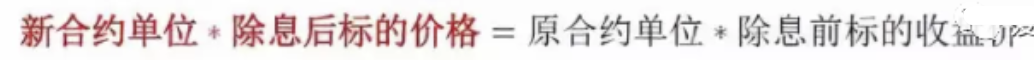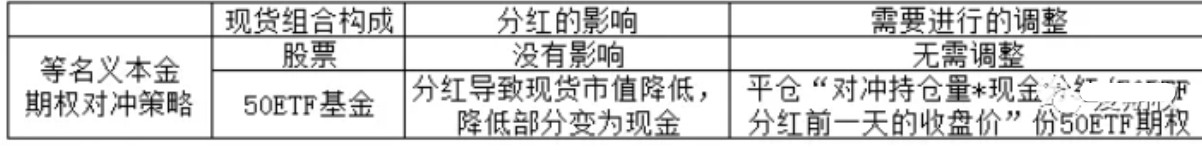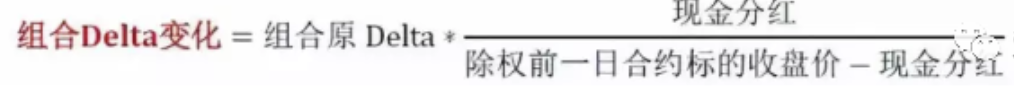FangQuant › Option

### The impact of ETF dividend on risk hedging with options

submitted 2020-01-01 22:28:39

"Huaxia SSE 50ETF" will deliver dividend and be ex-dividend on December 2, 2019. On that day, 50ETF options will be adjusted accordingly, and the exercise price, contract unit and unit premium will be changed. What impact will these changes have on risk hedging? We will explain in this article:

(I) equal nominal principal hedging

There are two definitions of "notional principal", i.e. "exercise price * contract unit" and "underlying price * contract unit". Generally, hedging transactions need to balance the value of long-short portfolio as much as possible. The second definition is more conducive to this state. Therefore, hedging traders generally use " underlying price * contract unit" to define notional principal.

The ex-dividend adjustment of 50ETF on December 2, 2019 only includes dividend, and does not involve share allotment. According to the option rules, on December 2, 2019, the adjustment method of the contract unit is as follows:

New Contract Unit = Old Contract Unit * underlying closing price before ex-dividend day/( underlying closing price before ex-dividend day-cash dividend)

In other words:

New underlying price* New Contract Unit= Old closing price *Old Contract Unit

The left and right sides of the equal sign are the corresponding nominal value of the contract before and after the adjustment, which shows that the nominal value is not affected by the adjustment. Therefore, if the common stock portfolio is hedged with options in accordance with the principle of "equal nominal value", the transaction will not be affected by the adjustment of option contract in December 2, 2019.It should be noted that if the spot hedged by options is a 50ETF fund, the dividend will lead to the decrease of the spot market value and the increase of cash, resulting in a relatively higher nominal value of the option contract. In order to maintain consistency, some options contracts need to be closed.

In general, for the option adjustment of December 2, 2019, under the "equal nominal value" hedge mode, if the spot portfolio is stocks, then no operation is required; if the spot is 50ETF fund, then some option contracts need to be closed. See the following table for specific operation methods:

 Spot position Dividend impact Adjustment needed equal nominal principal hedging option strategy Stocks No impact No need for adjustment 50 ETF fund Lead spot market value decrease, the part of decrease is changed to cash Close “hedging unit * cash dividend/ underlying closing price before ex-dividend day” share 50 ETF”

In addition, for two kinds of special spot construction modes: copy SSE50 index and copy 50ETF purchase application form, because the market value of 50ETF dividend will not be affected, so there is no difference from operation of common stock portfolio, and no adjustment is needed.

(II) Fixed delta hedging

For hedging transactions with fixed delta value, that is, to keep the negative delta size of option portfolio constant, 50ETF will change the delta of option portfolio due to the change of contract exercise price and unit after ex dividend on December 2, 2019.Specifically, the delta of one option is calculated as follows (taking call option as an example):

Call Delta=Contract unit *N(d1)=contract unit *N[(ln(S/K)+(r+σ^2/2)*T)/( σ*T^0.5)]

On that day, the exercise price is adjusted as follows:

New execution price = Old execution price * (underlying closing price before ex-dividend day-cash dividend)/underlying closing price before ex-dividend day

This shows that the ratio of the subject price to the exercise price before and after the adjustment remains unchanged:

S/K=S’/K’

As the parameters such as interest rate, maturity time and volatility remain unchanged, so N(d1) remains unchanged, that is, the delta of each unit option contract remains unchanged. Therefore, the impact of 50ETF dividend on Delta is mainly caused by the contract unit. According to the rules, the new and old contract units have the following relationship:

New contract unit/Old contract unit-1= cash dividend/( underlying closing price before ex-dividend day-cash dividend)

The above results are equal to the increase proportion of contract Delta. Therefore, after 50 ETF dividend, the change amount of portfolio delta is:

Portfolio delta change=portfolio old delta* cash dividend/( underlying closing price before ex-dividend day-cash dividend)

Therefore, the adjustment of fixed delta hedging strategy after 50ETF dividend is as follows:

 Spot position Dividend impact Adjustment needed (both is ok) Fixed delta hedging Stock position Unit delta unchanged, but unit increase,| delta| increase (1)close option position “position * cash dividend / ( 50 ETF closing price before ex-dividend day - cash dividend)” share 50ETF option contract; (2) buy “old market value * cash dividend/(50 ETF closing price before ex-dividend day - cash dividend)” shares in original composition

50ETF分红对运用期权进行风险对冲的影响

“华夏上证50ETF”将于2019年12月2日分红除息，当天50ETF期权将发生相应调整，行权价、合约单位、单位权利金都会改变。这些变化会对风险对冲造成什么影响？我们在本文中予以说明：
（一）等名义本金对冲
“名义本金”的定义有两种，“行权价*合约单位”与“标的价格*合约单位”，通常情况下，对冲交易需要尽可能使多空组合的价值相平衡，第二种定义更有助于实现这种状态，因此，对冲交易者一般采用“标的价格*合约单位”来定义名义本金，本节同样采纳该定义。（二）固定Delta对冲2019.12.2当天，行权价的调整方式如下：